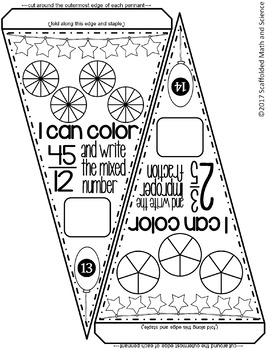# Improper Fractions and Mixed Numbers Pennant

Rated 4.86 out of 5, based on 112 reviews
112 Ratings;
4th - 6th
Subjects
Resource Type
Standards
Formats Included
• PDF
Pages
14 pages
Report this resource to TPT
##### Also included in
1. This bundle includes 6 sets of fractions pennants for comparing, adding, subtracting, multiplying, dividing and converting. Each set includes math pennants for students to color, an optional student answer sheet and answer keys.Math pennants are a fun way to show off student work. Students love seei
Price \$12.50Original Price \$21.00Save \$8.50

### Description

In this collaborative activity students convert improper fractions to mixed numbers and mixed numbers to improper fractions. Students also color portioned circles to show the fractions.

This is the same activity as Improper Fractions & Mixed Numbers Pennant {holiday themed}.

All pennants also include other places to color so that students can get creative. There are 13 pennants asking students to convert from improper fractions to mixed numbers and 12 pennants asking students to convert the other way. Once a pennant is complete, it can be hung along a string in your classroom to show the world that, "Hey, we know how to convert fractions!"

There are 2 pennants per page. All fraction pictures are partitioned and have denominators of 2, 3, 4, 5, 6, 8, 9, 10 and 12.

Included are 25 pennants with fraction conversion problems, an optional student answer sheet and an answer key. Each group of students can be given a set of pennants, crayons, scissors and either glue, tape or a stapler. Students can be in charge of cutting out the pennants. Groups can compete to see which group can complete the most pennants or your entire class can add pennants to one string as they complete each one.

You may also like:

Comparing Fraction Size Pennant

Fraction Pennants Bundle

Total Pages
14 pages
Included
Teaching Duration
N/A
Report this resource to TPT
Reported resources will be reviewed by our team. Report this resource to let us know if this resource violates TPT’s content guidelines.

### Standards

to see state-specific standards (only available in the US).
Explain why a fraction 𝘢/𝘣 is equivalent to a fraction (𝘯 × 𝘢)/(𝘯 × 𝘣) by using visual fraction models, with attention to how the number and size of the parts differ even though the two fractions themselves are the same size. Use this principle to recognize and generate equivalent fractions.
Add and subtract fractions with unlike denominators (including mixed numbers) by replacing given fractions with equivalent fractions in such a way as to produce an equivalent sum or difference of fractions with like denominators. For example, 2/3 + 5/4 = 8/12 + 15/12 = 23/12. (In general, 𝘢/𝘣 + 𝘤/𝘥 = (𝘢𝘥 + 𝘣𝘤)/𝘣𝘥.)